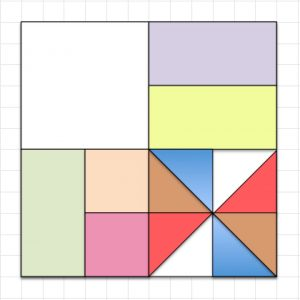The square is one unit and it is split into smaller parts. Copy the picture and label each part with the fraction it shows.

Which coloured bits would you use to show that two eighths equals one quarter? $\frac{2}{8}=\frac{1}{4}$

Which coloured bits would you use to show that two sixteenths equals one eighth? $\frac{2}{16}=\frac{1}{8}$

What can you say about the fractions shown by the triangles?

How many small pink squares give the same fraction as the three white bits taken together?

Write down fractions that add up to one half in different ways using the fractions shown in the diagram.

Draw your own pattern in a square and label the fractions in your pattern.

FRACTIONS BY HALVES GAME Copy the pattern onto thick card and draw a frame around it 1 cm wide. Use a knife to cut out the 14 pieces leaving the frame intact: $\frac{1}{4}$ (1 piece),   $\frac{1}{8}$ (3 pieces),  $\frac{1}{16}$ (2 pieces),  $\frac{1}{32}$  (8 pieces).

Put in a bag 14 identical cards with the fractions $\frac{1}{4}$  written on one card, $\frac{1}{8}$ written on 3 cards, $\frac{1}{16}$ on 2 cards and $\frac{1}{32}$  on 8 cards.

The game is for 2 players or 2 teams who add 2 fractions in the easy version and  3 fractions in the advanced version.

The first player randomly picks 2 cards, selects 2 puzzle pieces that match the fractions on those cards and places them in the frame, then adds the 2 fractions. The second player does the same and they do this alternately until there are no cards left.

For example, a green and a red piece make  $\frac{1}{8}+\frac{1}{32}=\frac{5}{12}$.    On each round the players must agree on the totals and the player with the largest total score wins a point.

For the 3-card version put a blank card in the bag to make 15 cards and a fair game.